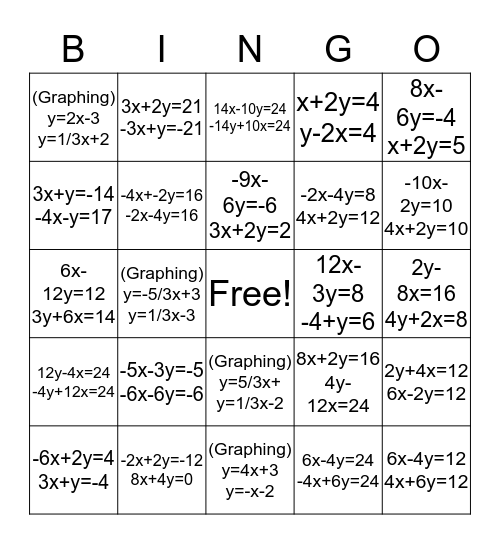# Chapter 3 BINGOThis bingo card has a free space and 24 words: -5x-3y=-5 -6x-6y=-6, -2x+2y=-12 8x+4y=0, -9x-6y=-6 3x+2y=2, -6x+2y=4 3x+y=-4, 8x-6y=-4 x+2y=5, (Graphing) y=2x-3 y=1/3x+2, 3x+y=-14 -4x-y=17, 3x+2y=21 -3x+y=-21, 12x-3y=8 -4+y=6, (Graphing) y=5/3x+ y=1/3x-2, -2x-4y=8 4x+2y=12, 6x-12y=12 3y+6x=14, 8x+2y=16 4y-12x=24, 2y+4x=12 6x-2y=12, -4x+-2y=16 -2x-4y=16, 6x-4y=24 -4x+6y=24, 2y-8x=16 4y+2x=8, 14x-10y=24 -14y+10x=24, -10x-2y=10 4x+2y=10, (Graphing) y=-5/3x+3 y=1/3x-3, 6x-4y=12 4x+6y=12, 12y-4x=24 -4y+12x=24, x+2y=4 y-2x=4 and (Graphing) y=4x+3 y=-x-2.

## Play Online